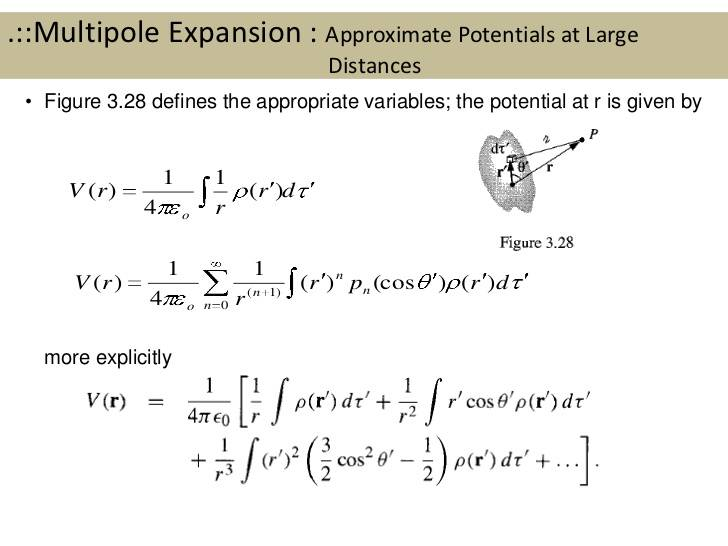# Multipole Expansion of a Thin rod

• sitkican

## Homework Statement

Consider a very thin rod lying on the z axis from z = −L/2 to z = L/2. It carries a uniform charge density λ. Show that away from the rod, at the point r (r >>L), the potential can be written as V (r, θ) = (2Lλ/4πε0)(1/L)[ 1 + 1/3(L/2r)2P2(cos θ) + 1/3(L/2r)4 P4(cos θ) + · · ·# (1)

## Homework Equations## The Attempt at a Solution

At the beginning, I tried to solve this question using cylindrical coordinates as there is some theta dependence in Legendre polynomial but it did not work.Then,I took the volume integral using cartesian coordinates and treating theta as constant.By doing that I could just take the Legendre polynomials out of the integration and calculate the integral with respect to z. I calculated the integral and put values of n up to n=4 and get the exact result in the square brackets but I have λL/4πε0r outside while I have to have 2λL/4πε0L.

What should I do know? Is my assumption,the theta is constant, true?